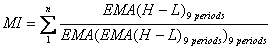Chart Pro online
User name (email):

 Recently used

 Forex

 Indices

# Mass Index (MI)

Mass Index (MI) is indicator in technical analysis developed to identify trend reversals by measuring range between high and low prices (narrowing or widening).

Mass index is calculated by formula:In calculation is used exponential moving average EMA applied to subtraction of high and low values. For period of EMA is used value of 9. For n parameter is usually used value of 25.Mass Index (MI)

Interpretation

When value of 25-period mass index rises above 27 and than falls below 26.5 it is signal for trend reversal.

Example